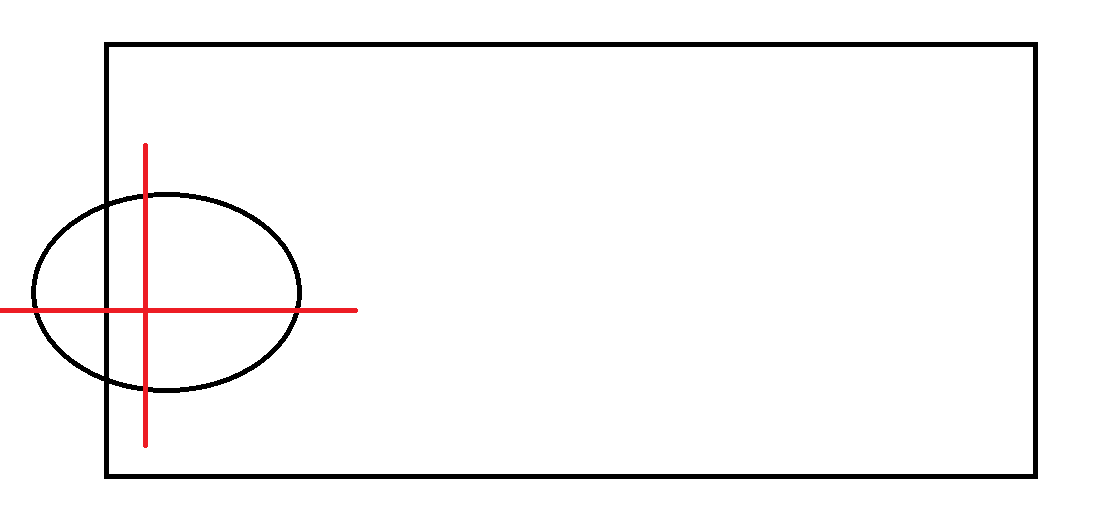# What does the x and y value mean here? Can you show in a figure?

``````function checkBoundaryCollision() {

if (x - ballRadius <= 0 || x + ballRadius >= canvasWidth) {

dx = -dx;

}

if (y - ballRadius <= 0 || y + ballRadius >= canvasHeight) {

dy = -dy;

}

}
``````Full code here: https://codepen.io/pelko/pen/gOjXdaq

The x and y values appear to be the co-ordinate positions of the centre of the ball (i.e. where the x and y axes of the red cross meet, x being horizontal and y being vertical).
So, the code you posted detects whether the edges of the ball meet (or cross) the boundaries of the canvas, by adding/subtracting the radius of the ball from the position of its centre.
If so, the direction of the ball in either axis (dx, dy) will change accordingly, causing the ball to ‘bounce’ off of the boundary in the opposite direction.

I only gave this a cursory glance but that what it seems to me… please correct me if I’m wrong!

1 Like